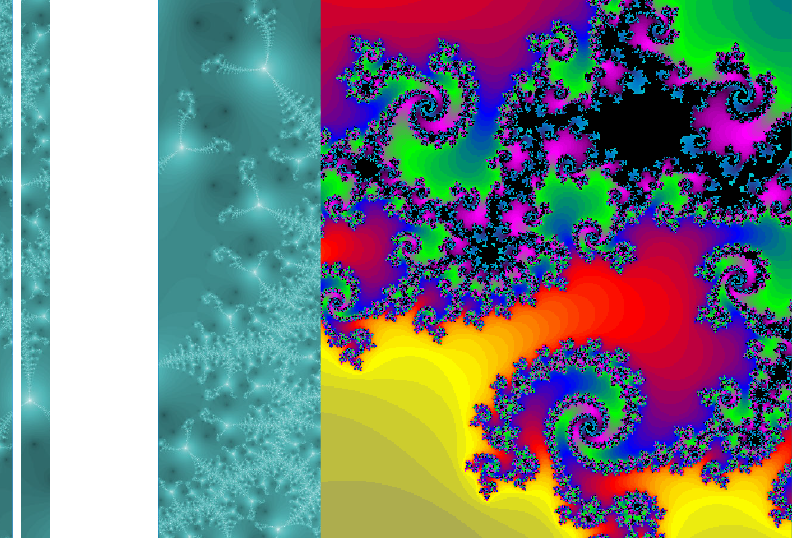Univerza na Primorskem Fakulteta za matematiko, naravoslovje in informacijske tehnologijeSI | EN
natisni

Korchmáros Gábor, Università della Basilicata, Italy.

Title: Symmetries of finite projective planes

Abstract: A finite projective plane is a pair \$(\cP;\cL)\$ where \$\cP\$ is a non empty set whose elements are called points and \$\cL\$ is a family of subsets of \$\cP\$ whose elements are called lines satisfying the three conditions, called incident axioms

• `any two distinct points are contained in exactly one line;`
• `any two distinct lines have exactly one common point;`
• `there exist three points non contained in the same line. `

Fundamental properties are: Each line consists of the same number of points, each point is contained in the same number of lines, and these two parameters coincide. As usual, this number is indicated by \$n+1\$ where \$n\ge 2\$ is an integer, called the order of the projective plane. The total number of points, as well as of lines, is \$n^2+n+1\$The classical example of a finite projective plane is the Galois plane \$PG(2,q)\$ of order \$q\$ arising from a finite field of order \$q=p^h\$, where \$p\$ is a prime number and \$h\geq 1\$ is an integer, by means of homogeneous coordinates.

A symmetry (usual called collineation) is a permutation on the pointset which takes lines to lines. The collineations of a projective plane form a group. For instance, the collineation group of \$PG(2,q)\$ is isomorphic to the semilinear group \$P\Gamma L(2,q)\$

Several important groups, such as the 3-dimensional projective linear group \$PGL(3,q)\$, the 3-dimensional projective unitary group \$PGU(3,q)\$, and the (simple) Suzuki group, are collineation groups of finite projective planes.

A general problem in finite geometry is to determine those pointsets in a projective plane which are left invariant by one of the above groups, or by some other interesting group. In many cases, such a pointset \$\Omega\$ has nice geometric features and the collineation group leaving \$\Omega\$ invariant has an interesting (sometimes, primitive and even doubly transitive) action on \$\Omega\$.

In this talk we show the most important such pointsets and also discuss related known results and open problems.